• 给出了闭区间[a，b]上为凸函数的f（x）的一个定积分的有界性质，并应用该性质对一类特殊的均值不等式进行了证明。
• 定义于区问1二[-1,1]上的实值函数f,若它的一切Lagrange插值多项式在BMO(1)范数下一致有界,则称f为完全BMO一有界函数。本文引人这一概念并讨论这类函数的性质
• 二元有界变差函数的Fourier级数的收敛性，吴伟燕，俞国华，设f(x, y)是对每个变量都是周期的函数, 在矩形区域上, f(x, y) 是Vitali意义下的有界变差函数, 首先证明f(x, y)的若干性质. 若f(x, y)属于（V）�
• 利用分析技巧得到了Post-Gamma算子一阶绝对矩量的渐近估计式，并结合区间分割技术和Bojanic-Cheng方法研究了Post-Gamma算子关于导函数为局部有界函数的点态逼近估计，同时得到了Post-Gamma算子的几何性质
• 函数的传统定义：设在某变化过程中有两个变量x、y，如果对于x在某一...函数的三种运算 函数的四则运算 复合运算 求逆运算函数的基本性质：单调性 有界性 奇偶性 周期性函数的近代定义：设A，B都是非空的数的集合，...
函数的传统定义：设在某变化过程中有两个变量x、y，如果对于x在某一范围内的每一个确定的值，y都有唯一确定的值与它对应，那么就称y是x的函数，x叫做自变量。我们将自变量x取值的集合叫做函数的定义域，和自变量x对应的y的值叫做函数值，函数值的集合叫做函数的值域。函数的三种运算 函数的四则运算 复合运算 求逆运算函数的基本性质：单调性 有界性  奇偶性 周期性函数的近代定义：设A，B都是非空的数的集合，f：x→y是从A到B的一个对应法则，那么从A到B的映射f：A→B就叫做函数，记作y=f(x)，其中x∈A，y∈B，原象集合A叫做函数f(x)的定义域，象集合C叫做函数f(x)的值域，显然有CB。符号y=f(x)即是“y是x的函数”的数学表示，应理解为：x是自变量，它是法则所施加的对象；f是对应法则，它可以是一个或几个解析式，可以是图象、表格，也可以是文字描述；y是自变量的函数，当x为允许的某一具体值时，相应的y值为与该自变量值对应的函数值，当f用解析式表示时，则解析式为函数解析式。y=f(x)仅仅是函数符号，不是表示“y等于f与x的乘积”，f(x)也不一定是解析式，在研究函数时，除用符号f(x)外，还常用g(x)，F(x)，G(x)等符号来表示。对函数概念的理解函数的两个定义本质是一致的，只是叙述概念的出发点不同，传统定义是从运动变化的观点出发，而近代定义是从集合、映射的观点出发。这样，就不难得知函数实质是从非空数集A到非空数集B的一个特殊的映射。由函数的近代定义可知，函数概念含有三个要素：定义域A、值域C和对应法则f。其中核心是对应法则f，它是函数关系的本质特征。y＝f（x）的意义是：y等于x在法则f下的对应值，而f是“对应”得以实现的方法和途径，是联系x与y的纽带，所以是函数的核心。至于用什么字母表示自变量、因变量和对应法则，这是无关紧要的。函数的定义域（即原象集合）是自变量x的取值范围，它是构成函数的一个不可缺少的组成部分。当函数的定义域及从定义域到值域的对应法则完全确定之后，函数的值域也就随之确定了。因此，定义域和对应法则为“y是x的函数”的两个基本条件，缺一不可。只有当两个函数的定义域和对应法则都分别相同时，这两个函数才是同一个函数，这就是说：1）定义域不同，两个函数也就不同；2）对应法则不同，两个函数也是不同的；3）即使是定义域和值域都分别相同的两个函数，它们也不一定是同一函数，因为函数的定义域和值域不能唯一地确定函数的对应法则。例如：函数y＝x＋1与y＝2x＋1，其定义域都是x∈R，值域都为y∈R。也就是说，这两个函数的定义域和值域相同，但它们的对应法则是不同的，因此不能说这两个函数是同一个函数。定义域A，值域C以及从A到C的对应法则f，称为函数的三要素。由于值域可由定义域和对应法则唯一确定。两个函数当且仅当定义域与对应法则分别相同时，才是同一函数。例如：在①y＝x与 ，② 与 ，③y＝x＋1与 ，④y＝x0与y＝1，⑤y＝｜x｜与 这五组函数中，只有⑤表示同一函数。f（x）与f（a）的区别与联系f（a）表示当x＝a时函数f（x）的值，是一个常量。而f（x）是自变量x的函数，在一般情况下，它是一个变量，f（a）是f（x）的一个特殊值。如一次函数f（x）＝3x＋4，当x＝8时，f（8）＝3×8＋4＝28是一常数。当法则所施加的对象与解析式中表述的对象不一致时，该解析式不能正确施加法则。比如f（x）＝x2＋1，左端是对x施加法则，右端也是关于x的解析式，这时此式是以x为自变量的函数的解析式；而对于f（x＋1）＝3x2＋2x＋1，左端表示对x＋1施加法则，右端是关于x的解析式，二者并不统一，这时此式既不是关于x的函数解析式，也不是关于x＋1的函数解析式。函数的定义域：定义：原象的集合A叫做函数y＝f(x)的定义域，即自变量的允许值范围。当函数用解析式给出时，定义域就是使式子有意义的自变量的允许值的集合。求定义域：求定义域的三种基本方法：一是依据函数解析式中所包含的运算（除法、开平方等）对自变量的制约要求，通过解不等式（组）求得定义域；二是依据确定函数y＝f（x）的对应法则f对作用对象的取值范围的制约要求，通过解不等式（组）求得定义域；三是根据问题的实际意义，规定自变量的取值范围，求得定义域。如果函数是由一些基本函数通过四则运算构成的，那么它的定义域是使各个部分都有意义的x值组成的集合。对含参数的函数求定义域（或已知定义域，求字母参数的取值范围）时，必须对参数的取值进行讨论。当函数由实际问题给出时，其定义域由实际问题确定。函数的值域： 定义：象的集合C（C B）叫做函数y＝f(x)的值域，即函数值的变化范围。求值域的基本方法：依据各类基本函数的值域，通过不等式的变换，确定函数值的取值范围，在这一过程中，充分利用函数图像的直观性，能有助于结论的得出和检验。从定义域出发，利用函数的单调性，是探求函数值域的通法复合函数也称为函数的函数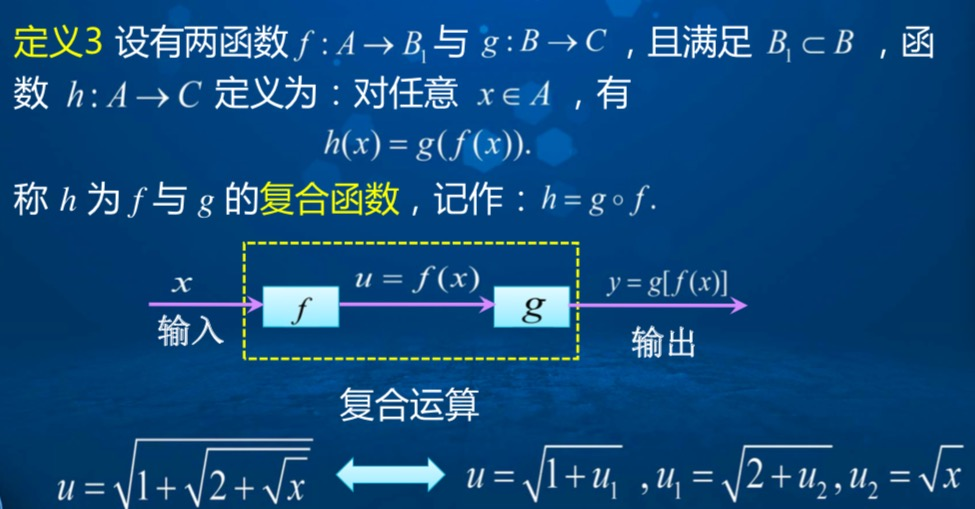反函数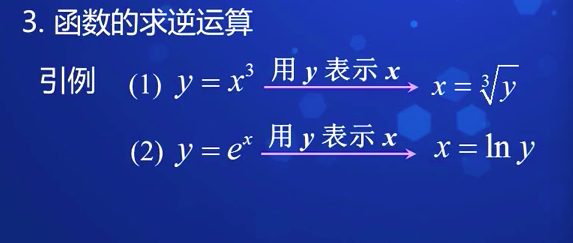集合的映射关系：
单射就是只能一对一，不能多对一
满射只要Y中的元素在X中都能找到原像就行了(一对一，多对一都行).
双射就是既是单射又是满射(一个对一个,每个都不漏掉).

反函数定义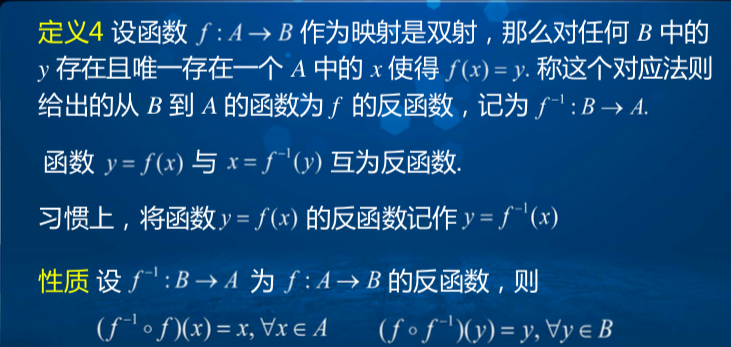转载于:https://www.cnblogs.com/jstong/p/8986877.html
展开全文• 文章目录闭区间连续函数的性质1.有界性定理2.最值定理3.零点存在定理4.介值定理5.一致连续定理(Cantor定理) 闭区间连续函数的性质 闭区间上的连续函数具有许多特殊的性质，在开区间上不一定具有。 1.有界性定理 命题...
文章目录闭区间连续函数的性质1.有界性定理2.最值定理3.零点存在定理4.介值定理5.一致连续定理(Cantor定理)
闭区间连续函数的性质
闭区间上的连续函数具有许多特殊的性质，在开区间上不一定具有。
1.有界性定理
命题：若函数$f(x)$在闭区间$[a,b]$上连续，则它在$[a,b]$上有界。
证明：用反证法。
如果$f(x)$在$[a,b]$上无界，则将$[a,b]$等分为两个小区间$[a,\dfrac{a+b}2],[\dfrac{a+b}{2},b]$，函数$f(x)$至少在其中一个小区间上无界，记作$[a_1,b_1]$；继续等分，函数依然至少在一个半区间上无界，记作$[a_2,b_2]$；依此进行，得到一个闭区间套$\{[a_n,b_n]\}$，这样就存在一个唯一的实数$\xi$属于所有区间$[a_n,b_n]$，且
$\xi=\lim_{n\to \infty}a_n=\lim_{n\to \infty}b_n.$
由于$f(\xi)$在$[a,b]$上连续，$f(x)$在$\xi$处连续，即$f(\xi)=\lim\limits_{x\to \xi}f(x)$存在，所以存在一个$\delta$，使得$f(x)$在$U^\circ(\xi,\delta)$有界（这是函数极限的有界性），结合函数的连续性，对于一切$x\in U(\xi,\delta)\cap [a,b]$存在
$|f(x)|\le M.$
由于闭区间套的长度趋近于0，所以一定存在一个$N$，使得$n>N$时，$b_n-a_n<\delta/2$，这就意味着
$[a_n,b_n]\sub U(\xi ,\delta),$
然而$f(x)$在$[a_n,b_n]$上无界，在$U(\xi,\delta)$上有界，这显然是矛盾的。
综上所述，闭区间上的连续函数有界。
开区间上连续函数无界的例子：$f(x)=\dfrac 1x$在$(0,1)$上无界。
2.最值定理
命题：若$f(x)$在闭区间$[a,b]$上连续，则它在$[a,b]$上必能取到最大值和最小值，即存在$\xi,\eta\in [a,b]$，对于一切$x\in [a,b]$，有$f(\xi)\le f(x)\le f(\eta)$。
证明：可以先找比最值稍弱的值——确界，再通过确界向最值逼近。
由有界性定理得$f(x)$在$[a,b]$上有界，所以$f(x)$的值域$R_f$存在下确界$\alpha=\inf R_f$和上确界$\beta =\sup R_f$。要证明存在$\xi\in [a,b]$使得$f(\xi)=\alpha$。
根据下确界的定义，一方面$f(x)\ge \alpha$，另一方面$\forall \epsilon>0,\exist x,f(x)<\alpha+\epsilon$，于是取$\{\epsilon_n\}$趋近于$0$，不妨设$\epsilon_n=\dfrac 1n$，由此得到数列$\{x_n\}$，其中$\alpha\le f(x_n)<\alpha+\frac 1n$。
由于$\{x_n\}$是一个有界数列，所以存在收敛子列$\{x_{n_k}\}$，由于$\{x_n\}$的构造方式，有
$\alpha\le f(x_{n_k})<\alpha+\frac 1{n_k}<\alpha+\frac 1k.$
两边同时关于$k$取极限，有$f(x_{n_{k}})\to \alpha$。不妨设$x_{n_{k}}\to x'$，那么由函数的连续性，有
$f(x')=f(\lim_{k\to \infty}x_{n_k})=\lim_{k\to \infty}f(x_{n_k})=\alpha,$
这就证明了$\exist x',f(x')=\alpha=\inf R_f$，证明了$f(x)$在$[a,b]$上取得到最小值。
同理构造收敛子列，可以证明$f(x)$在$[a,b]$上取得到最大值。
3.零点存在定理
命题：若函数$f(x)$在闭区间$[a,b]$上连续，且$f(a)f(b)<0$，则一定存在$\xi\in (a,b)$使得$f(\xi)=0$。
证明：要证明存在这样的$\xi$，关键是将其构造出来。
不失一般性，设$f(a)<0,f(b)>0$，可以定义集合$V=\{x|f(x)<0,x\in [a,b]\}$，显然集合$V$有界非空（至少含有点$a$），所以必有上确界，设为$\xi=\sup V$，现在想要证明$\xi\in (a,b),f(\xi)=0$。
先证明$\xi\in (a,b)$，由于$f(a)<0$，所以存在一个$\delta_1$使得$\forall x\in [a,a+\delta_1]$，$f(x)<0$；由于$f(b)>0$，所以存在一个$\delta_2$使得$\forall x\in [b-\delta_2,b]$，$f(x)>0$，这样就有$a+\delta_1\le \xi\le b-\delta_1$，所以$\xi\in(a,b)$。
取$x_n\in V,x_n\to \xi$，因为$f(x_n)<0$，所以$f(\xi)=f(\lim\limits_{n\to \infty}x_n)=\lim\limits_{n\to \infty}f(x_n)\le 0$，下证明$f(\xi)<0$是不可能存在的。
若$f(\xi)<0$，则根据$f(x)$在$\xi$的连续性，存在一个$\delta$，使得$[\xi-\delta,\xi+\delta]$内的$x$满足$f(x)<0$，这就与$\xi$是$V$的上确界矛盾（因为$\xi+\delta>\xi$且$f(\xi+\delta)<0$），所以必定有$f(\xi)=0$。
4.介值定理
命题：如果函数$f(x)$在闭区间$[a,b]$上连续，则它一定能取到最大值$M=\max\{f(x)|x\in [a,b]\}$和最小值$m=\min\{f(x)|x\in [a,b]\}$之间的任何一个值。
证明：建立介值定理与零点存在定理的联系。
由最值定理，存在$\xi,\eta\in [a,b]$使得$f(\xi)=m,f(\eta)=M$，不妨假设$\xi<\eta$。
对于任意中间值$C$即$m，考察辅助函数
$g(x)=f(x)-C,$
显然$g(x)$在$[a,b]$上也是连续函数，且$g(\xi)=m-C<0,g(\eta)=M-C>0$，由零点存在定理，必定有$\zeta\in (\xi,\eta)$，使得
$g(\zeta)=0=f(\zeta)-C,\quad f(\zeta)=C.$
这就证明了介值定理。同时，介值定理表明了$f(x)$在$[a,b]$上的值域为$R_f=[m,M]$。
5.一致连续定理(Cantor定理)
一致连续性：设$f(x)$定义在区间$X$上，$\forall \varepsilon>0,\exist \delta(\varepsilon)>0$，只要$|x_1-x_2|<\delta(x_1,x_2\in X)$，就有$|f(x_1)-f(x_2)|<\varepsilon$，就称$f(x)$在区间$X$上一致连续。
一致连续的充要条件：$f(x)$在$X$上一致连续，等价于对任何点列$\{x_n'\},\{x_n''\}(x_n',x_n''\in X)$，有
$\lim_{n\to \infty}(x_n'-x_n'')=0\Rightarrow \lim_{n\to \infty}(f(x_n')-f(x_n''))=0.$
命题(Cantor)：若函数$f(x)$在闭区间$[a,b]$上连续，则它在$[a,b]$上一致连续。
证明：采用反证法。
如果$f(x)$在$[a,b]$上不是一致连续的，则存在$\varepsilon_0>0$与点列$\{x_n'\},\{x_n''\}$满足
$|x_n'-x_n''|<\frac 1n,\quad |f(x_n')-f(x_n'')|\ge\varepsilon_0.$
由致密性定理，存在收敛的子列$\{x_{n_k}'\}$使得$\lim\limits_{k\to \infty}x_{n_k}'=\xi,\xi\in[a,b]$，在点列$\{x_n''\}$中选取同样下标的点列$\{x_{n_k}''\}$，则$|x_{n}'-x_{n_k}''|<\frac 1{n_k}$，且$|f(x_{n_k}')-f(x_{n_k}'')|\ge \varepsilon_0$，且
$\lim_{k\to \infty}x_{n_k}''=\lim_{k\to \infty}(x_{n_k}''-x_{n_k}')+\lim_{k\to \infty}x_{n_k}'=\xi.$
由于函数$f(x)$在$\xi$连续，所以
$\lim_{k\to \infty}f(x_{n_k}')=\lim_{k\to \infty}f(x_{n_k}'')=f(\xi).$
于是
$\lim_{k\to \infty}[f(x_{n_k}')-f(x_{n_k}'')]=0,$
这与$f(x_{n_k}')-f(x_{n_k}'')\ge \varepsilon_0$矛盾，因此一定是一致连续的。
该定理在开区间上不成立，是因为$\xi$可能取到区间的边界。


展开全文• 方法 利用有界变差函数的性质。结果 用有界变差函数的局部全变差来准确刻画三角插值多项式的Fejér和对有界变差函数的逼近结果。结论 给出三角插值多项式的Fejér和对ω-型有界变差函数的-致逼近估计。
• 主要讨论了正则的有界非...1内的上界问题,利用正则的有界非零函数的性质、极值原理和三角不等式,对正则的有界非零函数前五项系数和|a0+a1+a2+a3+a4|的上界进行估计,得到其上界一个新的表达式,从而推广了Krzyz猜测问题。
• 函数可积性的勒贝格判据指出，一个有界函数是黎曼可积的，当且仅当它的所有不连续点组成的集合测度为0。黎曼函数的不连续点集合即为有理数集，是可数的，故其测度为0，所以由勒贝格判据，它是黎曼可积的。数学
• 有界函数一定同时存在上下界，即有界⇔ 存在上下界。 二 函数的单调性 三 函数的奇偶性 奇函数图像关于原点对称 偶函数图像关于y轴对称 四 函数的周期性 常见的周期函数有sinx，...
一 函数的有界性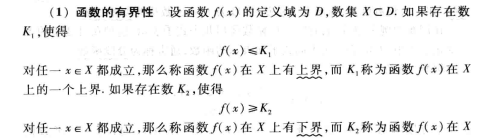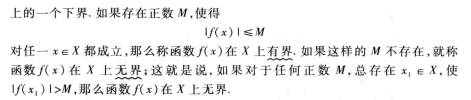有界函数一定同时存在上下界，即有界 ⇔ 存在上下界。

二 函数的单调性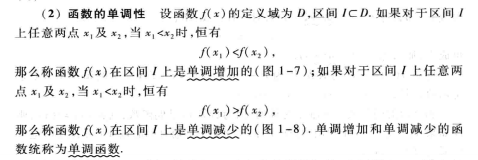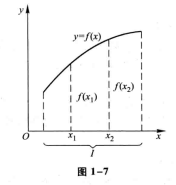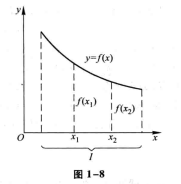三 函数的奇偶性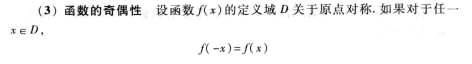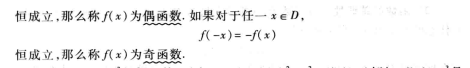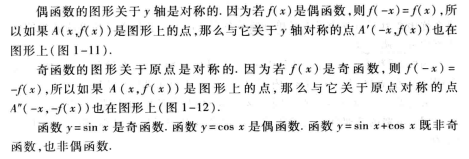奇函数图像关于原点对称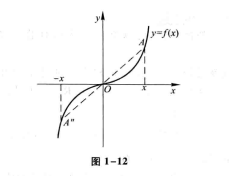偶函数图像关于y轴对称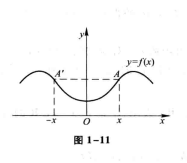奇，偶函数的运算

奇函数 ± 奇函数 = 奇函数。

偶函数 ± 偶函数 = 偶函数。

奇函数 * 奇函数 = 偶函数。

偶函数 * 偶函数 = 偶函数。

奇函数 * 偶函数 = 奇函数。

若f(x)为可导函数，若f(x)为奇函数，则f'(x)为偶函数。若f(x)为偶函数，则f'(x)为奇函数。

四 函数的周期性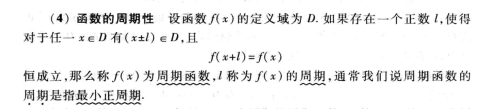常见的周期函数有sinx，cosx，tanx。其中sinx，cosx均为以2π为周期的周期函数，tanx则是以π为周期的周期函数。

展开全文周期性
• 局部有界性 f在x0处f在x_0处f在x0​处 连续⇒f在某U(x0)\Rightarrow f在某U(x_0)⇒f在某U(x0​) 有界 局部保号性 f在x0处f在x_0处f在x0​处 连续 f(x0)f(x_0)f(x0​)&gt;0&gt;0>0 ⟹\...
文章目录局部性质局部有界性局部保号性复合函数连续性闭区间性质有界性定理最大最小值定理介值性定理注意！！
局部性质
局部有界性
$f在x_0处$ 连续$\Rightarrow f在某U(x_0)$ 有界
局部保号性

$f在x_0处$ 连续

$f(x_0)$$>0$  $\Longrightarrow$
$\forall r
$f(x)>r$
\

$f在x_0处$ 连续

$f(x_0)$$<0$  $\Longrightarrow$
$\forall r>f(x_0),\exists 某U(x_0),使得对\forall x\in U(x_0)有$
$f(x)

也就是说，连续函数只要有一个点>或<0，就能得到一个小区间大于或小于0！！！
复合函数连续性

$f在x_0连续$
$g在u_0连续$
$u_0=f(x_0)\Rightarrow$$g。f在点x_0处连续$
以上结论可写为
$\lim\limits_{x\rightarrow x_0}g(f(x))=g(\lim\limits_{x\rightarrow x_0}f(x))=g(f(x_0))$

闭区间性质
有界性定理
$f$在闭区间$[a,b]$上连续$\Rightarrow f$在闭区间$[a,b]$上有界
最大最小值定理
$f$在闭区间上连续$\Rightarrow f$有最大最小值
介值性定理

$f$在闭区间$[a,b]$上连续
$f(a)\ne f(b)$
$\mu$在$f(a) and f(b)$之间$\Rightarrow 至少\exists 一点x_0\in(a,b)$，使得$f(x_0)=\mu$
应用介值性定理可推得连续函数的下述性质，这才是最经常应用到的！
$f$在闭区间$[a,b]$上连续
$m\le f(x)\le M$
$m<\xi $\Rightarrow 则至少\exists 一点x_0\in(a,b)$，使得$f(x_0)=\xi$

注意！！
这里的$x_0$在开区间$(a,b)$中，所以如果题里有出现“$\exists$ 一点$\xi \in(a,b)$，balabala…使得某个等式成立”，除了联想到拉屎、罗尔、柯西这些中值定理，还有这个介值定理啊啊啊！！！


展开全文• t∈R的性质，推出了指数有界C余弦算子函数的Taylor展开式，然后借助Petfis积分、Holder不等式及随机变量的矩生成函数等工具，得到了指数有界C余弦算子函数的概率逼近公式及Vonorovskaya型渐近公式。
• 研究特殊的有界形变函数空间SBD（Ω...首先利用泛函的一些性质，通过BD函数的近似可微性以及被积函数的特殊条件给出体积部分的积分表示，其次利用迹算子的连续性等，给出面积部分的积分表示，从而得到泛函的积分表示。
• 一、有界性与最大最小值定理 二、零点定理与介值定理 2.1、零点定理 2.2、介值定理 2.2.1、推论
• 基本初等函数的图像与性质 在数学的发展过程中，形成了最简单最常用的六类函数，即常数函数、幂函数、指数函数、对数函数...常数函数是有界函数，周期函数（没有最小的正周期）、偶函数； 常数函数既是单调增加函数又数学
• 若f(x)∈c[a,b]，则存在k,对任意x∈[a,b]有|f(x)|≤k，即函数在闭区间上连续，则函数在闭区间上有界 注解： 由定理1我们知道，函数在闭区间上连续，则函数在闭区间上可以取到最小值和最大值，即函数
• 基于测度和积分理论给出了随机变量条件特殊函数的定义...首先证明了条件特征函数的有界性、一致性和非负定性，然后给出了条件独立随机变量和的条件特征函数的算法，以及随机变量的各阶条件矩与条件特征函数之间的关系．
• 一 三角函数特殊角的函数值 二 连续，可导，存在原函数，函数有界之间的关系 ... 函数的极限具有唯一性，当函数在某一点取得的极限值为±∞时，表明函数在该点极限不存在，即函数在该点发散。 ...数学
• 利用Euclidean-Jordan代数将非线性互补问题(NCP)一类价值函数推广到对称锥互补问题(SCCP)上,并证明了SCCP等价于一个...此外,研究了使得价值函数具有全局误差界条件,并给出了使得价值函数水平集有界的一个较弱条件.
• 现在我们证明连续实值函数的一个重要性质，即有界定理。有界定理表明连续函数在紧集上是有界的并且在集合上的某些点取得最大值与最小值，准确的描述放到定理5中。为了理解上面的结论，我们考虑非紧集上函数会发生...
• 文章介绍了一种分母为二次仅基于函数值二元有理插值曲面，研究了这种插值曲面的有界性质和点控制方法；证明了在插值区域内，无论参数如何选择，插值函数值都是有界的，得到了该插值不依赖于参数估计表达式...
• 文章目录初探函数及其性质1 函数性质1.1 有界性1.2 单调性1.3 奇偶性1.4 周期性2 基本初等函数图像2.1 三角函数曲线图像2.2 幂函数2.3 指数函数与对数函数3. 复合函数4 初等函数 1 函数性质 1.1 有界性   在定义域...
• 本文将圆内有界函数的幂级数之部分和的有界性定理推广到Hp类函数，并在此基础上，讨论了稍广的一类函数的相应l司题。
• 视频03 函数的几个简单性质1 函数的有界函数的上界和下界s.t . f(x) &lt;= M V x 属于 I ， 则称M为区间I上的上界s.t . f(x) &lt;= N V x 属于 I ， 则称M为区间I上的下界若f(x) 在区间I上有界 &lt;==...
• 一般地，我们两种定义连续函数的性质。一种是利用ϵ\epsilonϵ-δ\deltaδ语言定义，一种是利用序列极限定义。在一元函数中，这两种定义是等价的。同时，利用分量连续的性质，不难证明这两种定义在多元函数中也是...
• 从这一结果出发证明了，当定义在（0，+∞）上的次可加函数与其自变量之比为有界函数时，次可加函数必存在上下确界函数，并证明了其上下确界函数均为齐次线性函数。进一步地，次可加数列和次可加函数的这些性质还可...
• 视频21四、连续函数在闭区间上的性质函数在区间I上的最大值和最小值定义设函数f(x) 在区间I 上定义如果x0 属于I，使得任意 x 属于 If(x0) &lt;= f(x) (或 f(x0) &gt;= f(x) ) 则称f(x0)是f(x)在区间I上的...高等数学
• 首先，考虑了非空紧子集X上共形迭代函数系{Si}i∞=0，如果它满足有界畸变性质和弱分离条件，那么|Si（X）|→0。其次，研究了两类特殊自相似无穷迭代函数系。其中一类中吸引子是唯一并且是紧，并运用加权......